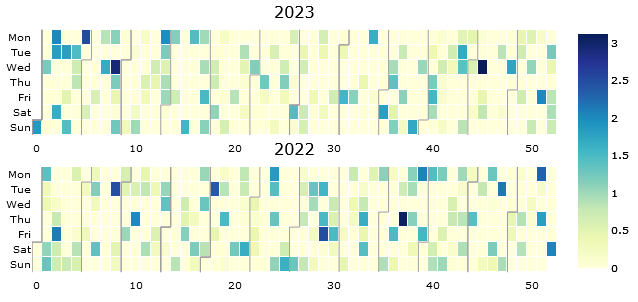# Plotly express figure (calplot) incorrectly displayed in Holoviz panel

I have a piece of code that produces a different plot in a jupyter notebook and a holoviz panel app.

First let us create some data:

``````    import pandas as pd
import numpy as np
from plotly_calplot import calplot
import panel as pn

allDays = pd.date_range('1/1/2022', periods=730, freq='D')
selectedDays = np.random.choice(allDays, 1000)
events = pd.Series(np.random.randn(len(selectedDays)), index=selectedDays)
df = pd.DataFrame(events, columns=["value"])
df["date"] = df.index
``````

Then I create the following figure:

``````    fig = calplot(
df,
x="date",
y="value",
years_title=True,
colorscale="YlGnBu",
).update_layout(
font_color="black",
plot_bgcolor="white",
).update_traces(
showscale = True,
selector=dict(type='heatmap'),
)
``````

If I now type `fig` in a jupyter notebook, the image looks like this:

However, if I type `pn.serve(fig)`, the image looks like this:That is, the months are not shown and week numbers are shown instead. How can I get the months? Also, is this a bug or a feature?